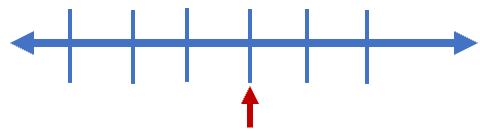# Math Worksheets Land

Math Worksheets For All Ages

# Math Worksheets Land

Math Worksheets For All Ages

# Fractions on a Number Line Worksheets

Helping students understand what a fraction actual is, at this level, is very challenging. We encourage teachers and students to use visual tools to help students understand the concept of parts and wholes. Using number lines is a great technique to help students grasp this concept quickly and more accurately. Number lines are horizontally drawn straight lines that extend infinitely in each direction. The values increase as you move across from left to right. The number lines often begin by be break up into as many parts that represent the parts in one whole value (denominator). We then make each segment by equal to one part of the whole (numerator). These worksheets are great way to show students the concept of a fraction by using a numbers line. Students learn how to create them from scratch and represent both numerators and denominators.

### Aligned Standard: Grade 3 Fractions - 3.NF.2

• Answer Keys - These are for all the unlocked materials above.

### Homework Sheets

The first one is just to get student comfortable with numbers lines again.

• Homework 1-Write a fraction to represent the point on the number line.
• Homework 2- Answer questions 1-5 as true or false using the number line above.
• Homework 3- Use the number line above to mark the following points.

### Practice Worksheets

There a great mix of variety in here. This makes sure that students are reading all the directions.

• Practice 1- Draw and mark a fraction on number line.
• Practice 2Draw two arrows on the numbers line to indicate the addition problem.
• Practice 3- Draw two arrows on the line to display the problem.
• Practice 4- Draw the sums on the number line.

### Math Skill Quizzes

You have to think of the whole number line as equal to 1 in some cases.

• Quiz 1- Draw the following points on the number line above.
• Quiz 2- Circle the correct answer below.

### How to Visually Represent a Fraction

To understand fractions, you need to represent them visually. There are multiple ways in which fractions can be represented. We have in the past, actually on the very last topic, applied the use of images in the form of visual fractions to better understand the nature of the parts and whole that are present in a fractional form.

If you were told this lesson is going to take a quarter of an hour, then you already know we are saying 15 minutes. There is 60 min in an hour and 1/4th of an hour is 15 min. So, no matter how far you go, you will always be able to find fractions around you. This concept could be understood better by students if it were presented in a slightly different manner.

The first method commonly used for bringing fractions to life, is the linear method. In the linear method, the numbers are shown in a line to show the fraction size. In a linear model, the length of the line is equally divided into spaced intervals. The fraction can be then shown with the help of another line, which covers the entire length. This is the method that we are focusing on in this topic. For example, if we wanted to bring the fraction 3/5 to life, we would create a numbers line that is composed of 5 parts. All we would need to do is point at the 3rd part to indicate 3/5. The diagram below illustrates this.The second method is called the Area method in which the area is represented in the form of a shape commonly a circle or rectangle. The fraction is shown in the form of division, where there are numerators and denominators. For instance, if we were to work with 3/4 where the numerator represents the selected portions, and the denominator represents the total number of divisions. The image below would show the numerator as the filled space below and the denominator is represented by all the boxes.Unlock all the answers, worksheets, homework, tests and more!
Save Tons of Time! Make My Life Easier Now

## Thanks and Don't Forget To Tell Your Friends!

I would appreciate everyone letting me know if you find any errors. I'm getting a little older these days and my eyes are going. Please contact me, to let me know. I'll fix it ASAP.